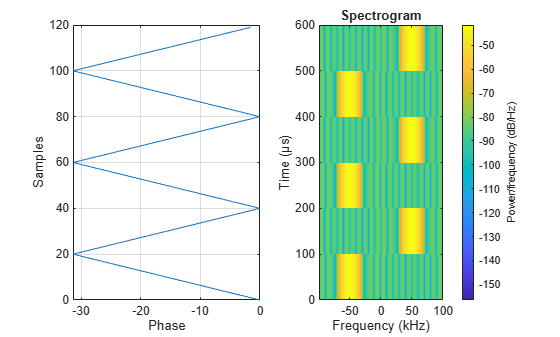# comm.FSKModulator

Modulate using M-ary FSK method

## Description

The `FSKModulator` object modulates using the M-ary frequency shift keying method. The output is a baseband representation of the modulated signal.

To modulate a signal using frequency shift keying:

1. Define and set up your FSK modulator object. See Construction.

2. Call `step` to modulate a signal according to the properties of `comm.FSKModulator`. The behavior of `step` is specific to each object in the toolbox.

Note

Starting in R2016b, instead of using the `step` method to perform the operation defined by the System object™, you can call the object with arguments, as if it were a function. For example, ```y = step(obj,x)``` and `y = obj(x)` perform equivalent operations.

## Construction

`H = comm.FSKModulator` creates a modulator System object, `H`. This object modulates the input signal using the M-ary frequency shift keying (M-FSK) method.

`H = comm.FSKModulator(Name,Value)` creates an M-FSK modulator object, `H`, with each specified property set to the specified value. You can specify additional name-value pair arguments in any order as (`Name1`,`Value1`,...,`NameN`,`ValueN`).

`H = comm.FSKModulator(M,FREQSEP,RS,Name,Value)` creates an M-FSK modulator object, `H`. This object has the `ModulationOrder` property set to `M`, the `FrequencySeparation` property set to `FREQSEP`, the `SymbolRate` property set to `RS`, and the other specified properties set to the specified values.

## Properties

 `ModulationOrder` Number of frequencies in modulated signal Specify the number of frequencies in the modulated signal as a numeric positive integer scalar value that is a power of two. The default is `8`. `BitInput` Assume bit inputs Specify whether the input is bits or integers. The default is `false`. When you set this property to `false`, the `step` method input requires a numeric (except single precision data type) column vector of integer values between `0` and `ModulationOrder`–1. In this case, the input vector can also be of data type logical if `ModulationOrder` equals `2`. When you set this property to `true`, the `step` method input requires a double-precision or logical data type column vector of bit values. The length of this vector is an integer multiple of log2(`ModulationOrder`). This vector contains bit representations of integers between `0` and `ModulationOrder`–1. `SymbolMapping` Symbol encoding Specify how the object maps an integer or group of log2(`ModulationOrder`) bits to the corresponding symbol as one of `Binary` | `Gray`. The default is `Gray`. When you set this property to `Gray`, the object uses Gray-coded ordering. When you set this property to `Binary`, the object uses natural binary-coded ordering. For either type of mapping, the object maps the lowest frequency to the integer 0 and maps the highest frequency to the integer M–1. In baseband simulation, the lowest frequency is the negative frequency with the largest absolute value. `FrequencySeparation` Frequency separation between successive tones Specify the frequency separation between successive tones in the modulated signal in Hertz as a positive, real scalar value. The default is `6` Hz. To avoid output signal aliasing, specify an output sampling rate, Fs = `SamplesPerSymbol`$×$`SymbolRate`, which is greater than `ModulationOrder` multiplied by `FrequencySeparation`. `ContinuousPhase` Phase continuity Specify if the phase of the output modulated signal is continuous or discontinuous. The default is `true`. When you set this property to `true`, the modulated signal maintains continuous phase even when its frequency changes. When you set this property to `false`, the modulated signal comprises portions of `ModulationOrder` sinusoids of different frequencies. In this case, a change in the input value can cause a discontinuous change in the phase of the modulated signal. `SamplesPerSymbol` Number of samples per output symbol Specify the number of output samples that the object produces for each integer or binary word in the input as a positive, integer scalar value. The default is `17`. `SymbolRate` Symbol duration Specify the symbol rate in symbols per second as a positive, double-precision, real scalar. The default is `100`. To avoid output signal aliasing, specify an output sampling rate, Fs = `SamplesPerSymbol`$×$`SymbolRate`, which is greater than `ModulationOrder` $×$ `FrequencySeparation`. The symbol duration remain the same, regardless of whether the input is bits or integers. `OutputDataType` Data type of output Specify the output data type as one of `double` | `single`. The default is `double`.

## Methods

 step Modulate using M-ary FSK method
Common to All System Objects
`release`

Allow System object property value changes

`reset`

Reset internal states of System object

## Examples

collapse all

Modulate and demodulate a signal using 8-FSK modulation with a frequency separation of 100 Hz.

Set the modulation order and frequency separation parameters.

```M = 8; freqSep = 100;```

Create FSK modulator and demodulator System objects™ with modulation order 8 and 100 Hz frequency separation.

```fskMod = comm.FSKModulator(M,freqSep); fskDemod = comm.FSKDemodulator(M,freqSep);```

Create an additive white Gaussian noise channel, where the noise is specified as a signal-to-noise ratio.

```ch = comm.AWGNChannel('NoiseMethod', ... 'Signal to noise ratio (SNR)','SNR',-2);```

Create an error rate calculator object.

`err = comm.ErrorRate;`

Transmit one hundred 50-symbol frames using 8-FSK in an AWGN channel.

```for counter = 1:100 data = randi([0 M-1],50,1); modSignal = step(fskMod,data); noisySignal = step(ch,modSignal); receivedData = step(fskDemod,noisySignal); errorStats = step(err,data,receivedData); end```

Display the error statistics.

```es = 'Error rate = %4.2e\nNumber of errors = %d\nNumber of symbols = %d\n'; fprintf(es,errorStats)```
```Error rate = 1.40e-02 Number of errors = 70 Number of symbols = 5000 ```

Visualize symbol mapping of an FSK modulated signal with a spectrogram.

Specify 20 samples for each symbol. 0 maps to -50 kHz (negative phase slope) and 1 maps to +50 kHz (positive phase slope).

```mod = comm.FSKModulator; mod.ModulationOrder = 2; mod.FrequencySeparation = 100000; mod.SamplesPerSymbol = 20; mod.SymbolMapping = 'Gray'; mod.SymbolRate = 1e4 ```
```mod = comm.FSKModulator with properties: ModulationOrder: 2 BitInput: false SymbolMapping: 'Gray' FrequencySeparation: 100000 ContinuousPhase: true SamplesPerSymbol: 20 SymbolRate: 10000 OutputDataType: 'double' ```
```x = mod([0 1 0 1 0 1]'); figure; subplot(1,2,1); plot(unwrap(angle(x)),0:length(x)-1); grid on; xlabel('Phase'); ylabel('Samples') subplot(1,2,2); spectrogram(x,20,0,[],mod.SymbolRate*mod.SamplesPerSymbol,'centered')```## Algorithms

This object implements the algorithm, inputs, and outputs described on the M-FSK Modulator Baseband block reference page. The object properties correspond to the block parameters, except: